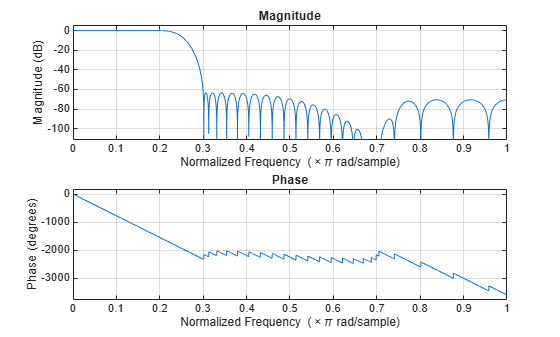# generateFilteringCode

Generate MATLAB code for a filter cascade

## Syntax

``generateFilteringCode(FC)``
``generateFilteringCode(FC,fileName)``

## Description

````generateFilteringCode(FC)` creates a MATLAB® function that contains code to create the stages of a filter cascade, `FC`, and calls each stage in sequence. If the filters in each stage support code generation, you can generate C/C++ code from the function returned by `generateFilteringCode`.```

example

````generateFilteringCode(FC,fileName)` generates code and saves the resulting function to the file specified in `fileName`.```

## Examples

collapse all

Design a two-stage decimator with a 100-Hz transition width, a 2-kHz sampling frequency, and 60-dB attenuation in the stopband. The decimator needs to downsample by a factor of 4.

```decimSpec = fdesign.decimator(4,'Nyquist',4,'Tw,Ast',100,60,2000); filtCasc = design(decimSpec,'multistage','SystemObject',true);```

Verify your design by using `fvtool`.

` info(filtCasc)`
```ans = 'Discrete-Time Filter Cascade ---------------------------- Number of stages: 2 Stage1: dsp.FIRDecimator ------- Discrete-Time FIR Multirate Filter (real) ----------------------------------------- Filter Structure : Direct-Form FIR Polyphase Decimator Decimation Factor : 2 Polyphase Length : 10 Filter Length : 19 Stable : Yes Linear Phase : Yes (Type 1) Arithmetic : double Stage2: dsp.FIRDecimator ------- Discrete-Time FIR Multirate Filter (real) ----------------------------------------- Filter Structure : Direct-Form FIR Polyphase Decimator Decimation Factor : 2 Polyphase Length : 18 Filter Length : 35 Stable : Yes Linear Phase : Yes (Type 1) Arithmetic : double ' ```
` fvtool(filtCasc)`Generate code to filter data using this design. You cannot generate C/C++ code from the `dsp.FilterCascade` object directly, but you can generate C/C++ code from the generated function. The function defines the filter stages and calls them in sequence. The function is saved in a file called my`Decimator.m` in the current directory.

` generateFilteringCode(filtCasc,'myDecimator');`

The my`Decimator` function creates a filter cascade and calls each stage object in turn.

` type myDecimator`
```function y = myDecimator(x) %MYDECIMATOR Construct filter cascade and process each stage % MATLAB Code % Generated by MATLAB(R) 9.8 and DSP System Toolbox 9.10. % Generated on: 29-Feb-2020 01:07:14 % To generate C/C++ code from this function use the codegen command. % Type 'help codegen' for more information. %#codegen %% Construction persistent filter1 filter2 if isempty(filter1) filter1 = dsp.FIRDecimator( ... 'Numerator', [0.0021878514650437845 0 -0.010189095418136306 0 0.031140395225498115 0 -0.082785931644222821 0 0.30979571849010851 0.5 0.30979571849010851 0 -0.082785931644222821 0 0.031140395225498115 0 -0.010189095418136306 0 0.0021878514650437845]); filter2 = dsp.FIRDecimator( ... 'Numerator', [0.0011555011750488237 0 -0.0027482166351233102 0 0.0057681982289523072 0 -0.010736374060960912 0 0.018592020073668478 0 -0.031093723586671229 0 0.052603914610235683 0 -0.099130756073130377 0 0.31592697826202448 0.5 0.31592697826202448 0 -0.099130756073130377 0 0.052603914610235683 0 -0.031093723586671229 0 0.018592020073668478 0 -0.010736374060960912 0 0.0057681982289523072 0 -0.0027482166351233102 0 0.0011555011750488237]); end %% Process y1 = filter1( x ); y = filter2( y1); ```

## Input Arguments

collapse all

Filter cascade, specified as a `dsp.FilterCascade` System object.

File name where the generated function is saved, specified as a character vector or string scalar.

Data Types: `char` | `string`

Watch now